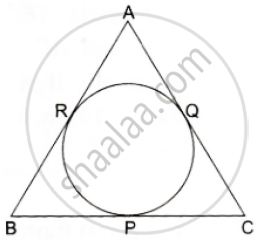# In the Given Figure, an Isosceles Triangle Abc, with Ab = Ac, Circumscribes a Circle. Prove that Point of Contact P Bisects the Base Bc. - Mathematics

In the given figure, an isosceles triangle ABC, with AB = AC, circumscribes a circle. Prove that point of contact P bisects the base BC.#### Solution

We know that tangent segments to a circle from the same external point are congruent
Now, we have
AR = AO, BR = BP and CP = CQ
Now, AB = AC
⇒ AR+ RB= AQ+ QC
⇒ AR + RB = AR + OC
⇒ RB  = QC
⇒  BP = CP
Hence, P bisects BC at P.

Concept: Concept of Circle - Centre, Radius, Diameter, Arc, Sector, Chord, Segment, Semicircle, Circumference, Interior and Exterior, Concentric Circles
Is there an error in this question or solution?